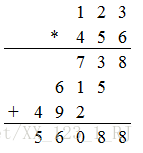# Python: LeetCode 43 Multiply Strings

Problem description:

43. String multiplication

Given two non-negative integers that are represented as strings, `num1` and `num2`, return the product of `num1` and `num2`, which is also represented as a string.

Example 1:

Input: num1 = “2”, num2 = “3”
output: “6”

Example 2:

Input: num1 = “123”, num2 = “456”
output: “56088”

Description:
`num1` and `num2` have lengths less than 110. `num1` and `num2` only contain the Numbers `0-9`. `num1` and `num2` do not begin with zero, unless it is the number 0 itself. cannot be processed using any of the standard library’s large number types (such as BigInteger) or by converting the input directly to an integer.
Problem analysis:
So this isn’t a very difficult problem, but if you just do the regular multiplication, you can figure it out. Figure below:The basic idea is as follows:
(1) can first flip the string, that is, start from the low order calculation.
(2) use an array to maintain the final result, updating it every time you multiply it, but pay attention to the carry case () pay attention to the two points, the same bit to add the carry, from low to high multiplication is carried. )
(3) finally, the `0` in front of the array is discarded and converted to string output.
Python3 implementation:

``````# @Time   :2018/08/05
# @Author :LiuYinxing
# String Num

class Solution:
def multiply(self, num1, num2):

res =  * (len(num1) + len(num2))  # Initialization, array to hold the product.
pos = len(res) - 1

for n1 in reversed(num1):
tempPos = pos
for n2 in reversed(num2):
res[tempPos] += int(n1) * int(n2)
res[tempPos - 1] += res[tempPos] // 10  # Offset
res[tempPos] %= 10  # fractional remainder
tempPos -= 1
pos -= 1

st = 0
while st < len(res) - 1 and res[st] == 0:  # How many zeros are in front of a statistic?
st += 1
return ''.join(map(str, res[st:])) # Remove the 0, then turn it into a string, and return

if __name__ == '__main__':
num1, num2 = "123", "456"
solu = Solution()
print(solu.multiply(num1, num2))``````

Welcome to correct me.